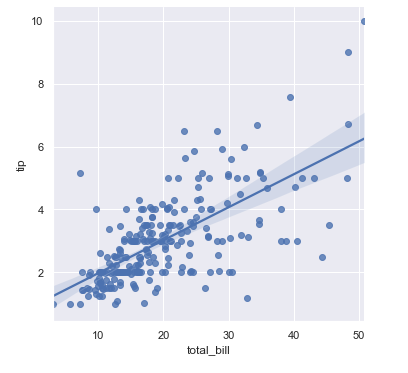# Seaborn Scatter Plot using scatterplot()- Tutorial for Beginners## Introduction

Scatter Plot is considered to be the most common and useful visualization for data exploration in data science and machine learning. In this article, we will go through the tutorial of the seaborn scatter plot for beginners. We will see various examples of creating different types of scatter plots using the scatterplot() function of the Seaborn library. So let’s start this tutorial.

## Seaborn Scatter Plot Tutorial

Technically speaking, Scatter Plot shows the relationship between two x and y, in most cases through such scatter plots, we can find out whether two variables are positively related or negatively related.

scatterplot() function in the Seaborn library uses a number of parameters, some of them are crucial to producing the visualization. In the following section, we’ll look at the syntax of scatterplot() along with the explanation for parameters

### Syntax for Seaborn Scatter Plot Function : scatterplot()

The following is the syntax of the scatter plot function.

seaborn.scatterplot(*, x=None, y=None, hue=None, style=None, size=None, data=None, palette=None, hue_order=None, hue_norm=None, sizes=None, size_order=None, size_norm=None, markers=True, style_order=None, x_bins=None, y_bins=None, units=None, estimator=None, ci=95, n_boot=1000, alpha=None, x_jitter=None, y_jitter=None, legend=’auto’, ax=None, kwargs)**

Parameters Info:

x, y : names of variables in data or vector data, optional

``````Here we pass the input data which is generally numeric.
``````

hue : name of variables in data or vector data, optional

``````In this parameter, we are mapping the colors for different variables
``````

size : name of variables in data or vector data, optional

``````Here, variables are grouped for producing points of varied sizes.
``````

style : name of variables in data or vector data, optional

``````In this parameter, variables are grouped to produce markers of various styles.
``````

data : DataFrame

``````Through this parameter, we pass the data for creating the scatter plot.
``````

palette : palette name, list, or dict, optional

``````With this parameter, we pass the colors for plot.
``````

markers : boolean, list, or dictionary, optional

``````In this parameter, we can specify the shape for markers.
``````

ci : int or “sd” or None, optional

``````This parameter sets the confidence interval, it can either take integer, standard deviation or none as values.
``````

alpha : float

``````This parameters specifies the opacity of the visualization.
``````

legend : “brief”, “full”, or False, optional

``````This parameter describes how we can define the legends for a plot.
``````

ax : matplotlib Axes, optional

``````Here we specify the axes on which plot is built.
``````

kwargs : key, value mappings

``This parameter defines the other keyword arguments.``

First, we load the seaborn library and then we load the dataset. Seaborn has a collection of datasets that will be used for building scatter plots.

In :
```import seaborn as sns
```
In :
```tips = sns.load_dataset("tips")
```

Output:

total_bill tip sex smoker day time size
0 16.99 1.01 Female No Sun Dinner 2
1 10.34 1.66 Male No Sun Dinner 3
2 21.01 3.50 Male No Sun Dinner 3
3 23.68 3.31 Male No Sun Dinner 2
4 24.59 3.61 Female No Sun Dinner 4

### 1st Example – Simple Seaborn Scatter Plot using scatterplot()

In this 1st example, we are using ‘tips’ dataset of Seaborn to create the simple scatter plot with the scatterplot() function of Seaborn

Here we pass three parameters to the scatterplot function. One of them is data, other two are the variables for the plot.

In :
```sns.scatterplot(data=tips, x="total_bill", y="tip", palette="winter_r")
```
Output: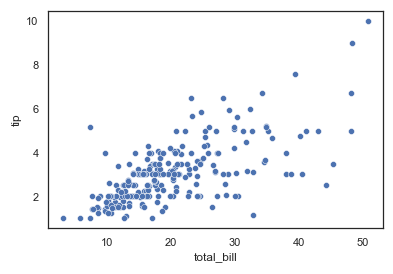### 2nd Example – Seaborn Scatter Plot with Hue

This 2nd example shows the use of hue variable, this helps in classifying the markers into different categories.

In the below example, we are categorizing the markers on the basis of the time attribute

In :
```sns.scatterplot(data=tips, x="total_bill", y="tip", hue="time", palette="winter_r")
```
Output: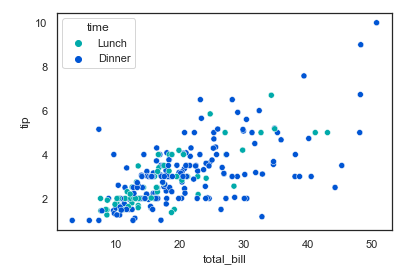### 3rd Example – Changing Marker Style of Scatter Plot

For the third example, we will be changing the style of the marker using style parameter of scatterplot().

In :
```sns.scatterplot(data=tips, x="total_bill", y="tip", hue="time", style="time", palette = "twilight")
```
Output: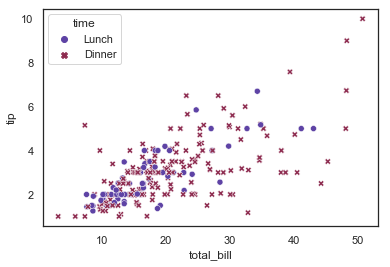### 4th Example – Multiple Categorization of Markers

Here in this instance, we are using both hue and style for plotting the scatter plot. The values passed to both the parameters are different in this case.

In :
```sns.scatterplot(data=tips, x="total_bill", y="tip", hue="day", style="time")
```
Output: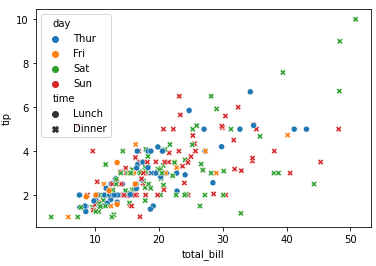### 5th Example – Using Numerical Attribute in Hue

In this example, we are passing a numerical attribute to the hue parameter. This will produce a quantitative semantic mapping with a color palette that has the same color with lighter to darker shades.

If the variable assigned to hue is numeric, the semantic mapping will be quantitative and use a different default palette

In :
```sns.scatterplot(data=tips, x="total_bill", y="tip", hue="size")
```
Output: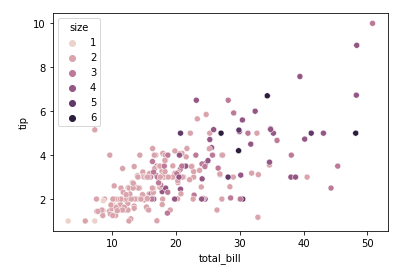### 6th Example – Scatterplot Marker Sizes and Hues using Seaborn relplot()

In this example, we are using a different dataset. To plot this kind of seaborn scatter plot that has varying sizes of markers based on the values, we are using relplot function. It takes x, y variables along with hue, size, sizes, alpha, palette, height, and data as parameters.

In :
```sns.set_theme(style="white")

# Load the example mpg dataset

# Plot miles per gallon against horsepower with other semantics
sns.relplot(x="horsepower", y="mpg", hue="origin", size="weight",
sizes=(40, 400), alpha=.5, palette="winter_r",
height=6, data=mpg)
```
Output: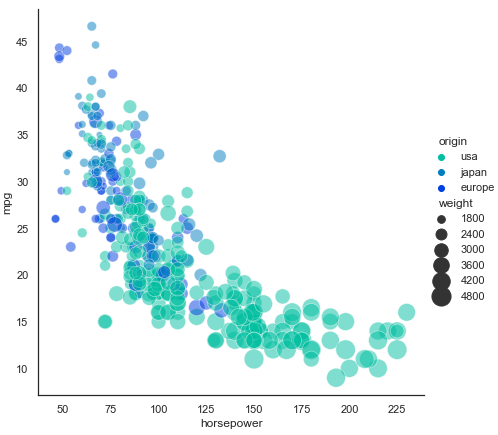### 7th Example – Seaborn Scatter Plot with Linear Regression Line using lmplot()

The regression line helps to visualize trends in the scatter plot. To plot such a visualization, we use lmplot() function of seaborn.

For this plot, we are using lmplot() function. With the help of this function, we can plot a scatter plot along with a regression line that shows perfectly fitted data.

In :
```import numpy as np
import matplotlib as plt

sns.set(color_codes=True)
np.random.seed(sum(map(ord, "regression")))